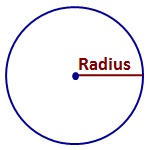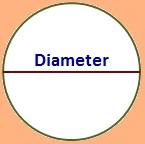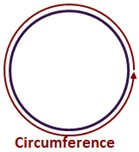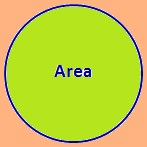©Ms. Garcia Math Name: ID: Quiz: Grade: Circle problems π (Pi) = 3.14 3.1 3 ? 1)a What is the diameter? yd b What is the circumference? yd c What is the area? yd² 2)a What is the radius ? cm b What is the circumference? cm c What is the area? cm² 3)a What is the diameter? m b What is the radius? m c What is the area? m² 4)a What is the radius? in b What is the diameter? in c What is the circumference? in